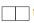# Chapter 8: Iteration and recursion - Online Test

Q1. In iteration, the loop body is repeatedly executed as long as the loop condition is
Explaination / Solution:
No Explaination.

Q2. Recursion must have
Explaination / Solution:
No Explaination.

Q3. An invariant for the loop body is known as a
Explaination / Solution:
No Explaination.

Q4. A loop invariant is true at
Explaination / Solution:
No Explaination.

Q5. A loop invariant need not be true
Explaination / Solution:
No Explaination.

Q6. Each time the loop body is executed, the variables
Explaination / Solution:
No Explaination.

Q7. A property of the variables which remains unchanged by the execution of the loop body. This unchanging property is called
Explaination / Solution:
No Explaination.

Q8. A loop invariant need not be true
Explaination / Solution:
No Explaination.

Q9. We wish to cover a chessboard withdominoes, the number of black squares and the number of white squares covered by dominoes, respectively, placing a domino can be modeled by
Explaination / Solution:
No Explaination.

Q10. If m x a + n x b is an invariant for the assignment a, b : = a + 8, b + 7, the values of m and n are
Explaination / Solution:
No Explaination.